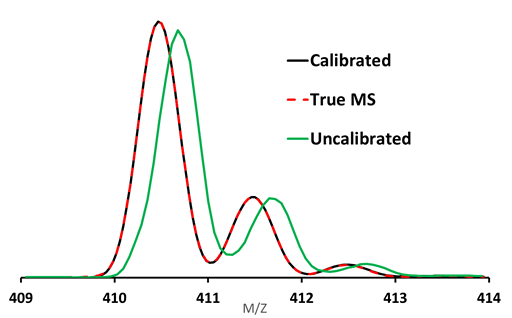# CLIPS™

CLIPS (Calibrated Line-shape Isotope Profile Search) is an entirely new and revolutionary way to attain fast and reliable elemental composition determination. Elemental composition is determined using the accurate mass information to identify a list of formula candidates. But even with very high mass accuracy, the list of formula candidates can make unambiguous formula determination difficult. Unlike approaches that use only mass accuracy for this purpose, CLIPS adds another accurate dimension to formula ID by matching the full isotope envelope of the unknown to the true mass spectrum of a candidate ion. In the past, this has not been possible due to the unknown line shape in mass spectrometry. The TrueCal™ implemented in Cerno software product including the MassWorks™ not only calibrates these instruments to accurate mass, it also calibrates the actual instrument line shape to a known mathematical function. This allows for the accurate calculation of the theoretical isotope profile for each formula candidate using the same line shape as the calibrated line shape and thus its true mass spectrum. The CLIPS algorithm then matches each formula candidate to the unknown and calculates a highly reliable statistic with typically less than 1% relative spectral error, a level of profile accuracy called Spectral Accuracy necessary to differentiate candidate formulas and arrive at unambiguous formula determination, even on a unit mass resolution system such as a single quadrupole MS.
The CLIPS algorithm first uses conventional formula determination based on mass accuracy and various formula search criteria (e.g. mass error or tolerance, elements, DBE, electron state, and charge). Once this list is calculated, CLIPS will then calculate the true mass spectrum for each formula and match it over the defined mass range to the unknown ion. The “Spectral Accuracy” of the match is then generated which is calculated as follows:

(1-RMSE)*100

where RMSE is the root mean squared error expressed as a fraction of the overall MS signal in the mass range. Thus, a RMSE of .005 would indicate that the fit spectral error is .005 or .5%; this translates to an easily interpretable Spectral Accuracy value of 99.5%.

CLIPS is included in all versions of MassWorks and other Cerno products.

### Ideal for unit resolution instruments (e.g. single quad GC/MS, LC/MS)

• 100x improvement in mass accuracy from 0.x to 0.00x Da
• Greater than 99% Spectral Accuracy achievable on chromatographic time scale
• Accurate formula ID enabled even at the unit resolution price
• Powerful mixture analysis with un-resolved MS signals

For more information and a sample application see:The figure above shows the high Spectral Accuracy match from CLIPS with TrueCal.